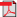# Search Results

1. P. Lassila and J. Virtamo, Importance Sampling for Monte Carlo Simulation of Loss Systems, COST257, TD(98)49, 1998(bib)
Abstract: In this paper we consider the use of traditional Monte Carlo simulation techniques for estimating very small blocking probabilities in multiservice loss systems. We derive an efficient sampling distribution, which has a composite form consisting of a weighted combination of exponentially twisted distributions. In the literature the composite distribution has been shown to be asymptotically efficient in systems such as the multiservice loss system. However, the asymptotic theory leaves open the question of how to choose the weights. For that we give heuristics to minimize the variance of the estimator by making the likelihood ratio as constant as possible in the set of the blocking states.